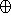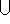## CGAL::approximated_offset_2

#include <CGAL/approximated_offset_2.h>

template<class Kernel, class Container>
typename Gps_circle_segment_traits_2<Kernel>::Polygon_with_holes_2
approximated_offset_2 ( Polygon_2<Kernel, Container> P, typename Kernel::FT r, double eps)
Provides a guaranteed approximation of the offset of the given polygon P by a given radius r - namely, the function computes the the Minkowski sum PBr, where Br is a disc of radius r centered at the origin. The function actually outputs a set S that contains the Minkowski sum, such that the approximation error is bounded by eps. Note that as the input polygon not be convex, its offset may not be a simple polygon. The result is therefore represented as a polygon with holes, whose edges are either line segments or circular arcs.
 Precondition: P is a simple polygon.

template<class Kernel, class Container, class DecompositionStrategy>
typename Gps_circle_segment_traits_2<Kernel>::Polygon_with_holes_2
 approximated_offset_2 ( Polygon_2 P, typename Kernel::FT r, double eps, DecompositionStrategy decomp)
Provides a guaranteed approximation of the offset of the given polygon P by a radius r, as described above. If the input polygon P is not convex, the function decomposes it into convex sub-polygons P1, ..., Pk and computes the union of the sub-offsets (namelyi(PiBr)). The decomposition is performed using the given decomposition strategy decomp, which must be an instance of a class that models the concept PolygonConvexDecomposition.
 Precondition: P is a simple polygon.